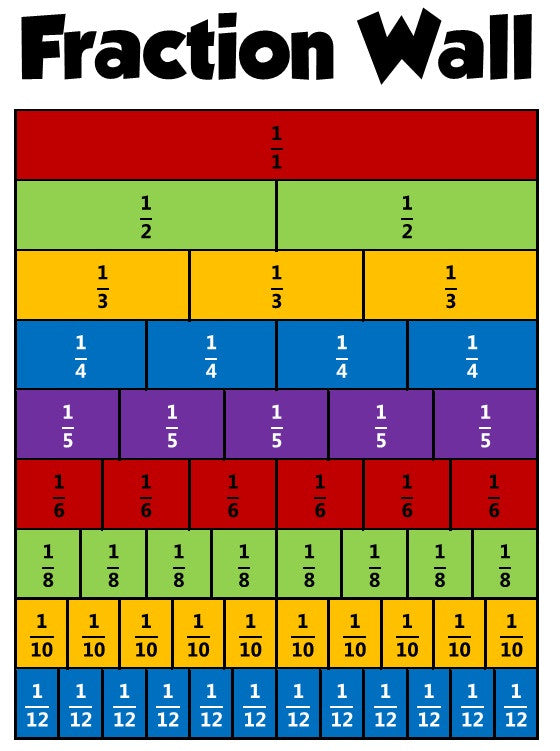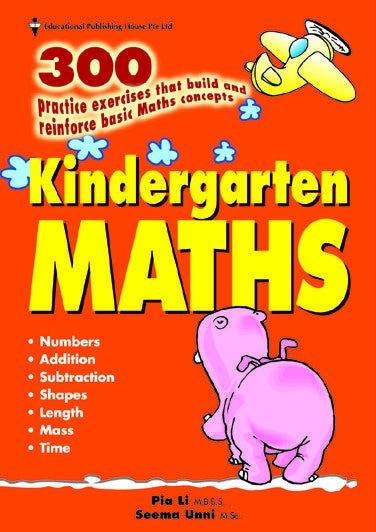Bringing Singapore Education Worldwide
We ship from Australia

# Singapore Books News — Maths

## Why are fractions so difficult to learn?

Fractions MathsAs many teachers and parents know, learning the various fraction operations can be difficult for many children. It's not the concept of a fraction that is difficult - it is the various operations: addition, subtraction, multiplication, division, comparing, simplifying, etc. of fractions And the simple reason why learning those operations proves difficult for many students is the way they are typically taught. Just look at the amount of rules there are to learn about fractions! 1. Fraction addition - common denominators Add the numerators, and use the common denominator 2. Fraction addition - different denominators First find a common denominator...

## The Mean, Median and Mode

Maths mean Median ModeAre you confused with what mean, median and mode means? This video will explain it and make you remember. Firm your kid's foundation in Maths with our maths series.